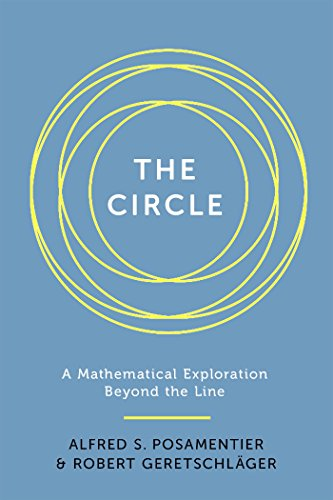## MATHS BITE: Ford Circles

A ford circle is a circle with centre, and radiuswhere p and q are coprime integers.

Notice that each Ford Circle is tangent to to the horizontal axis and any two Ford circles are either tangent or disjoint. The latter statement can be proven by finding the squared distance d^2 between the centres of the circles with (p,q) and (p’,q’) as the pairs of coprime integers.Let s be the sum of the radii:ThenHowever, we have thatand so, thus the distance between circles is greater or equal to the sum of the radii of the circles. There is equality iffIn this case, the circles are tangent to one another.

#### Total area of Ford Circles

(taken from Wikipedia)

As no two ford circles intersect, it follows immediately that the total area of the Ford circles:is less than 1.

From the definition, the area isSimplifying this expression gives usnoting that the last equality is given by considering the Dirichlet generating function for Euler’s totient function φ(q).

Given that ζ(4) = π^4/90, we getM x

## Nine-Point Circle

The nine-point circle is a circle that can be that can be constructed for any given triangle. It is named the nine-point circle as it passes through nine points defined from the triangle:

• The midpoint of each side of the triangle (Ma, Mb, Mc);
• The foot of each altitude (Ha, Hb, Hc);
• The midpoint of the line segment from each vertex of the triangle to point where the three altitudes meet, i.e. the orthocentre H, (Ea, Eb, Ec).

Note that for an acute triangle, six of the points – the midpoints and altitude feet – lie on the triangle itself. On the contrary, for an obtuse triangle two of the altitudes have feet outside the triangle, but these feet still belong to the nine-point triangle.

The nine-point circle is the complement to the circumcircle, which is the unique circle that passes through each of the triangle’s three vertices.

Although credited for its discovery, Karl Wilhelm Feuerbach did not entirely discover the nine-point circle, but rather the six point circle, as he only recognised that the midpoints of the three sides of the triangle and the feet of the altitudes of that triangle lay on the circle. It was mathematician Olry Terquem who was the first to recognise the added significance of the three midpoints between the triangle’s vertices and the orthocenter.

### Three Properties of the Nine-Point Triangle

• The radius of a triangle’s circumcircle is twice the radius of the same triangle’s nine-point circle.
• A nine-point circle bisects a line segment going from the corresponding triangle’s orthocenter to any point on its circumcircle.
• All triangles inscribed in a given circle and having the same orthocenter have the same nine-point circle.

Want to know more?

Click here to find out to construct a nine-point circle and here to read a quick proof of its existence!

M x

## New Books in Maths: October 2016 (II)

The Circle: A Mathematical Exploration Beyond the Line – Alfred S. Posamentier and Robert GeretschlagerThe circle has played a pivotal role in our society, appearing in our art, literature, and culture and has helped develop our industrial civilisation. So, it is no surprise that the circle has fascinated mathematicians since the ancient times.

This book aims to underline the many intriguing properties of the circle to beginners by describing how the circle “manifests itself in the field of geometry”, leading to important relationships and theorems. It also highlights how the circle continues to charm mathematicians today, as they continue to discover new and surprising properties about this most fundamental of shapes.

Elements of Mathematics: From Euclid to Gödel – John Stillwell

Elements of Mathematics takes readers on a journey of elementary mathematics, which as Stillwell shows is not as simple as one may believe. As he examines elementary mathematics from a 21st century standpoint, the beauty and scope of the discipline, as well as its limits, are revealed.

From Gaussian integers to propositional logic, Stillwell delves into arithmetic, computation, algebra, geometry, calculus, combinatorics, probability, and logic.” He describes how the research in these areas helped build the foundations of, and hence develop, modern mathematics into what it is today.

This is not Stillwell’s first book; apart from being a professor of mathematics at the University of San Francisco he has also written many books including ‘Mathematics and Its History’ and ‘Roads to Infinity’.

For those of you interested in the history of mathematics, this is a must read!

“An accessible read. . . . Stillwell breaks down the basics, providing both historical and practical perspectives from arithmetic to infinity.” – Gemma Tarlach, Discover

Read part 1 here! M x